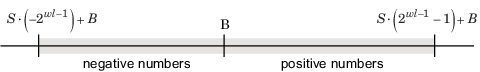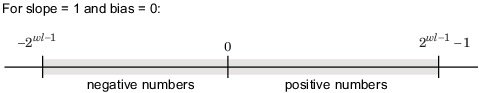Main Content

## Precision and Range

Note

You must pay attention to the precision and range of the fixed-point data types and scalings you choose in order to know whether rounding methods will be invoked or if overflows or underflows will occur.

### Range

The range is the span of numbers that a fixed-point data type and scaling can represent. The range of representable numbers for a two's complement fixed-point number of word length $wl$, scaling $S$ and bias $B$ is illustrated below:For both signed and unsigned fixed-point numbers of any data type, the number of different bit patterns is 2wl.

For example, in two's complement, negative numbers must be represented as well as zero, so the maximum value is 2wl -1 – 1. Because there is only one representation for zero, there are an unequal number of positive and negative numbers. This means there is a representation for $-{2}^{wl-1}$ but not for ${2}^{wl-1}$:#### Overflow Handling

Because a fixed-point data type represents numbers within a finite range, overflows and underflows can occur if the result of an operation is larger or smaller than the numbers in that range.

Fixed-Point Designer™ software allows you to either saturate or wrap overflows. Saturation represents positive overflows as the largest positive number in the range being used, and negative overflows as the largest negative number in the range being used. Wrapping uses modulo arithmetic to cast an overflow back into the representable range of the data type.

When you create a `fi` object, any overflows are saturated. The `OverflowAction` property of the default fimath is `saturate`. You can log overflows and underflows by setting the `LoggingMode` property of the `fipref` object to `on`.

### Precision

The precision of a fixed-point number is the difference between successive values representable by its data type and scaling, which is equal to the value of its least significant bit. The value of the least significant bit, and therefore the precision of the number, is determined by the number of fractional bits. A fixed-point value can be represented to within half of the precision of its data type and scaling.

For example, a fixed-point representation with four bits to the right of the binary point has a precision of 2-4 or 0.0625, which is the value of its least significant bit. Any number within the range of this data type and scaling can be represented to within (2-4)/2 or 0.03125, which is half the precision. This is an example of representing a number with finite precision.

#### Rounding Methods

When you represent numbers with finite precision, not every number in the available range can be represented exactly. If a number cannot be represented exactly by the specified data type and scaling, a rounding method is used to cast the value to a representable number. Although precision is always lost in the rounding operation, the cost of the operation and the amount of bias that is introduced depends on the rounding method itself. To provide you with greater flexibility in the trade-off between cost and bias, Fixed-Point Designer software currently supports the following rounding methods:

• `Ceiling` rounds to the closest representable number in the direction of positive infinity.

• `Convergent` rounds to the closest representable number. In the case of a tie, `convergent` rounds to the nearest even number. This is the least biased rounding method provided by the toolbox.

• `Zero` rounds to the closest representable number in the direction of zero.

• `Floor`, which is equivalent to two's complement truncation, rounds to the closest representable number in the direction of negative infinity.

• `Nearest` rounds to the closest representable number. In the case of a tie, `nearest` rounds to the closest representable number in the direction of positive infinity. This rounding method is the default for `fi` object creation and `fi` arithmetic.

• `Round` rounds to the closest representable number. In the case of a tie, the `round` method rounds:

• Positive numbers to the closest representable number in the direction of positive infinity.

• Negative numbers to the closest representable number in the direction of negative infinity.

Choosing a Rounding Method.  Each rounding method has a set of inherent properties. Depending on the requirements of your design, these properties could make the rounding method more or less desirable to you. By knowing the requirements of your design and understanding the properties of each rounding method, you can determine which is the best fit for your needs. The most important properties to consider are:

• Cost — Independent of the hardware being used, how much processing expense does the rounding method require?

• Low — The method requires few processing cycles.

• Moderate — The method requires a moderate number of processing cycles.

• High — The method requires more processing cycles.

Note

The cost estimates provided here are hardware independent. Some processors have rounding modes built-in, so consider carefully the hardware you are using before calculating the true cost of each rounding mode.

• Bias — What is the expected value of the rounded values minus the original values: $Ε\left(\stackrel{^}{\theta }-\theta \right)$?

• $Ε\left(\stackrel{^}{\theta }-\theta \right)<0$ — The rounding method introduces a negative bias.

• $Ε\left(\stackrel{^}{\theta }-\theta \right)=0$ — The rounding method is unbiased.

• $Ε\left(\stackrel{^}{\theta }-\theta \right)>0$ — The rounding method introduces a positive bias.

The following table shows a comparison of the different rounding methods available in the Fixed-Point Designer product.

Fixed-Point Designer Rounding ModeCostBias
`Ceiling`LowLarge positive
`Convergent`HighUnbiased
`Zero`Low
• Large positive for negative samples

• Unbiased for samples with evenly distributed positive and negative values

• Large negative for positive samples

`Floor`LowLarge negative
`Nearest`ModerateSmall positive
`Round`High
• Small negative for negative samples

• Unbiased for samples with evenly distributed positive and negative values

• Small positive for positive samples

`Simplest`
(Simulink® only)
LowDepends on the operation

## SupportGet trial now